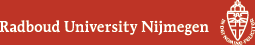## Geometry Seminar - Abstracts

### Talk

Wednesday 6 June 2018, 16:00-17:00 in HG03.085
Alexander Varchenko (University of North Carolina)
Hyperelliptic integrals modulo p and Cartier-Manin matrices

### Abstract

The hypergeometric solutions of the Knizhnik-Zamolodchikov (KZ) equations were constructed almost 30 years ago. The polynomial solutions of the KZ equations over the finite field Fp with a prime number p of elements were constructed recently. I will consider the example of the KZ equations whose hypergeometric solutions are given by hyperelliptic integrals of genus g. It is known that in this case the total 2g-dimensional space of holomorphic solutions is given by the hyperelliptic integrals. It turns out that the recent construction of the polynomial solutions over the field Fp in this case gives only a g-dimensional space of solutions, that is, a "half" of what the complex analytic construction gives. It turns out that all the constructed polynomial solutions over the field Fp can be obtained by reduction modulo p of a single distinguished hypergeometric solution. The corresponding formulas involve the entries of the Cartier-Manin matrix of the hyperelliptic curve.

(Back to geometry seminar schedule)